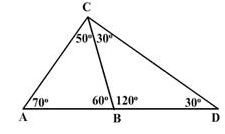# Test: Construction Of Triangles

## 10 Questions MCQ Test Mathematics (Maths) Class 9 | Test: Construction Of Triangles

Description
This mock test of Test: Construction Of Triangles for Class 9 helps you for every Class 9 entrance exam. This contains 10 Multiple Choice Questions for Class 9 Test: Construction Of Triangles (mcq) to study with solutions a complete question bank. The solved questions answers in this Test: Construction Of Triangles quiz give you a good mix of easy questions and tough questions. Class 9 students definitely take this Test: Construction Of Triangles exercise for a better result in the exam. You can find other Test: Construction Of Triangles extra questions, long questions & short questions for Class 9 on EduRev as well by searching above.
QUESTION: 1

Solution:
QUESTION: 2

Solution:
QUESTION: 3

### Which of these triangles are possible to construct by knowing only its altitude?

Solution:
QUESTION: 4

The construction of a triangle ABC, given that BC = 6 cm, B = ∠45° is not possible when difference of AB and AC is equal to :​

Solution:

Given, BC = 6 cm and ∠B= 45°
We know that, the construction of a triangle is not possible, if sum of two sides is less than or equal to the third side of the triangle.
i.e., AB + BC < AC
⇒ BC < AC – AB
⇒  6 < AC-AB
So, if AC – AB= 6.9 cm, then construction of ΔABC with given conditions is not possible.

QUESTION: 5

Choose the correct statement

Solution:
QUESTION: 6

The point of concurrence of the three angle bisectors of a triangle, is called

Solution: The point of interection of
medians - centroid
altitude - orthocentre
angle bisector - incentre
perpendicular bisectors - circumcentre
QUESTION: 7

In triangle ABC, side AB is produced to D so that BD = BC. If angle B = 60° and angle A = 70°, thenSolution:
in this particular given problem angle
corresponding to the sides
CDcd : angle CAB
as, angle ACD > angle CAB, So
QUESTION: 8

The point of intersection of the perpendicular bisectors of the sides of a triangle is called

Solution:
The perpendicular bisectors of the sides of a triangle intersect at a point called the circumcenter of the triangle, which is equidistant from the vertices of the triangle.
QUESTION: 9

To construct a right triangle whose base is 12 cm and sum of its hypotenuse and other side is 18 cm. We draw line segment AB of 12 cm. Draw a ray AX making 90° with AB. The next step is:

Solution:

The below given steps will be followed to construct the required triangle.

Step I:
Draw line segment AB of 12 cm. Draw a ray AX making 90deg with AB.
Step II:
Cut a line segment AD of 18 cm (as the sum of the other two sides is 18) from ray AX.
Step III:
Join DB and make an angle DBY equal to ADB.
Step IV:
Let BY intersect AX at C. Join AC, BC.

ΔABC is the required triangle.

QUESTION: 10

The lengths of the sides of some triangles are given, which of them is not a right angled triangle?

Solution: If a triangle is right angle then it must satisfy Pythagoras theorem

Now let us check whether all options satisfy it or not....

a) 5^2+12^2=169

5^2+12^2=13^2

b) 5^2+8^2 is not equal to 10^2

c) 3^2+4^2=5^2

d) 7^2+24^2=25^2

It is clear that 2nd option doesn't satisfy Pythagoras theorem...soo option 2 is the answer